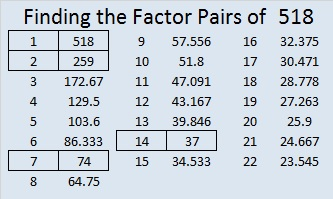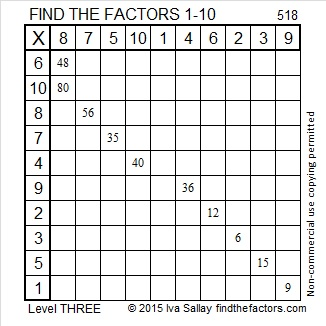# 518 and Level 3

Finding something interesting about the number 518 was as easy as 1-2-3.

518 = (5^1) + (1^2) + (8^3). Thank you stetson.edu for that fun fact.

518 is also the hypotenuse of the Pythagorean triple 168-490-518. Can you find the greatest common factors of those three numbers?Print the puzzles or type the solution on this excel file: 10 Factors 2015-06-08

—————————————————————————————————

• 518 is a composite number.
• Prime factorization: 518 = 2 x 7 x 37
• The exponents in the prime factorization are 1, 1, and 1. Adding one to each and multiplying we get (1 + 1)(1 + 1)(1 + 1) = 2 x 2 x 2 = 8. Therefore 518 has exactly 8 factors.
• Factors of 518: 1, 2, 7, 14, 37, 74, 259, 518
• Factor pairs: 518 = 1 x 518, 2 x 259, 7 x 74, or 14 x 37
• 518 has no square factors that allow its square root to be simplified. √518 ≈ 22.759613—————————————————————————————————

A Logical Approach to solve a FIND THE FACTORS puzzle: Find the column or row with two clues and find their common factor. Write the corresponding factors in the factor column (1st column) and factor row (top row).  Because this is a level three puzzle, you have now written a factor at the top of the factor column. Continue to work from the top of the factor column to the bottom, finding factors and filling in the factor column and the factor row one cell at a time as you go.